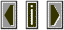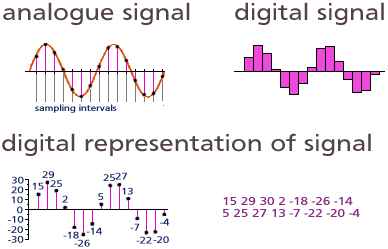Digital Representation of SoundThe representation of data in binary form.  The representation of continuous signals as a series of discrete values.  The discrete representation of sound waves.

 This is achieved by converting the sound energy by means of a transducer (such as a microphone) into analogous electrical variations of current. An analog to digital converter (ADC) is then used to periodically take a series of "snapshots" of the voltage level of the the analogous signal. This results in a data stream of numbers which can be processed and stored in computer memory or some other storage media and retrieved later to reconstruct the waveform using a digital to analog converter (DAC).The rate at which the "snapshots" of the signal level are taken is known as the sampling rate.

 Sampling rate determines the bandwidth of the resulting digital representation with a rate of twice the highest frequency desired being required.

 So, to represent the human range of hearing we would need to sample the sound at least 40,000 times per second. The current standard for digital reproduction of sound using Compact Discs involves a sampling rate of 44.1 kHz resulting in a theoretical bandwidth of 0 to 22,500 Hz.

 The size of the digital "word" used to encode the voltage levels of the analog signal give an indication of the dynamic range of the resulting digital representation as well as its signal to noise characteristics. The CD format uses a 16 bit format which allows for 65,536 discrete voltage steps to be represented with a resulting theoretical signal to noise ratio of approximately 96 dB.
Contrast with analog represention of sound.